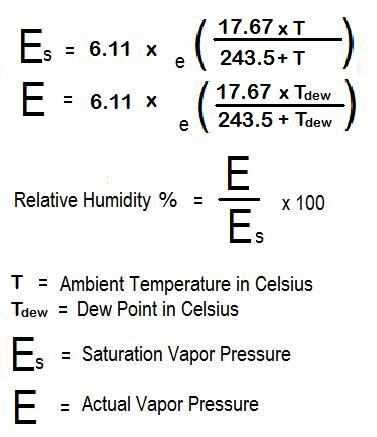CalcuNation

# Relative Humidity Calculator

## Calculate the relative humidity from temperature and dew point with this Humidity Calculator. Assuming a temperature range between -45 and 60 degrees celsius and no changes in air pressure.

Temp (Celsius):
Dew Point (Celsius):
Fill in the temperature and dew point to determine the approximate
relative humidity.

### What is Relative Humidity?

Relative humidity is the amount of water vapor in the air.

To calculate humidity use this Relative Humidity Formula:Example: When the ambient temperature (in celsius) is 35 degrees,
dew point is 30 degrees, the approximate relative humidity is:

Approximate Relative Humidity = 75%

### \$100 Promotion

Win \$100 towards teaching supplies! We want to see your websites and blogs.Enter Here

### Calculator Popups

Scientific Calculator
Simple Calculator

### Calculator Ideas

We use your calculator ideas to create new and useful online calculators.
Submit Calculator Idea